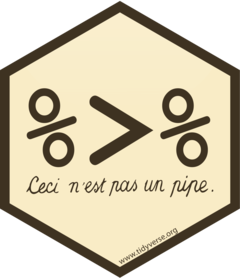# Part 6 The `%>%` forward-pipe operator

Let’s review the steps from the previous hands-on:

``````df_w2 <- pivot_longer(df_w,
cols=c("Weight_day0", "Weight_day10"),
values_to="weight",
names_to="day"
)
df_w3 <- separate(df_w2, col = "day",
sep="_",
into=c(NA, "day")
)``````

If we are only interested in the final tidy data frame (and not in the intermediate object `df_w2`), there is a simple and clean way to combine all steps.

The %>% (forward-pipe) operator from the `magritrrr` package (the `tidyverse` packages automatically load this operator):The pipe allows to process the output of a function as the input of the following function: it makes the code easier to read and understand:

• It pipes the output of a function as the first argument of the next function (from left to right):
• `mytibble %>% function1` is equivalent to `function1(mytibble)`
• `mytibble %>% function2(y)` is equivalent to `function1(mytibble, y)`
• `mytibble %>% function1 %>% function2` is equivalent to `function2(function1(mytibble))`

If we go back to the previous exercise, we can simplify the code the following way:

``````df_w_clean <- pivot_longer(df_w,
cols=c("Weight_day0", "Weight_day10"),
values_to="weight",
names_to="day") %>%
separate(col = "day",
into=c(NA, "day"))

# This is equivalent to:
df_w_clean <- df_w %>% pivot_longer(cols=c("Weight_day0", "Weight_day10"),
values_to="weight",
names_to="day") %>%
separate(col = "day",
into=c(NA, "day"))``````

#### HANDS-ON

Simplify the following piece of code using the `%>%` operator:

``````table5_1 <- separate(data=table5, col=rate, into=c("cases", "population"), sep="/")
table5_2 <- unite(data=table5_1, col=year, c("century", "year"), sep="")``````
``````table5_1 <- table5 %>%